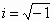# Translations of Encyclopedia about Mathematics

## Chaos

Chaos as we generally understand it has nothing to do with the mathematical concept of chaos.

A typical example of chaos in a seemingly deterministic system (a system where cause and effect but not arbitrariness play a role) is developments on the stock exchange, changes the weather, a sudden traffic jam or the behaviour of quantum particles in physics.

Mathematical chaos describes complex dynamic systems. These time dependent systems often behave quite sensitively and visibly chaotically to the degree that they are unforeseeable; this though while the individual components of the system are subject to explicit and natural laws.

Since the 1970s, mathematics began to study chaotic systems. Non-linear equations serve to make computations in such systems. An important process when making such calculations is the fact that certain computational procedures continually repeat themselves, where the attained value is inserted into the original value in the same equation and the result of the equation used once again… Something similar to if we had to extract the root of 16, then 4, and then once again 2… This repeated use of the result as the original value in a certain computational procedure is referred to as iteration.

The physicist Mitchell Feigenbaum discovered definite similarities between the patterns and structures of systems inclined towards chaos and derived from it certain quantities which he called Feigenbaum’s constants :

If we take a look at Feigenbaum’s diagram, we can recognise definite and continually repeating types of tree branches, which we refer to as bifurcation and which can be calculated with a computer.

There are three characteristics of chaotic systems:

Sensitivity

Chaotic systems react very sensitively to original values. In short, one can say that the waving of a butterfly’s wings is sufficient to fundamentally change a dynamic weather system. Small initial causes can have extreme effects.

Periodicity

A dynamic system shows similar behaviour in defined time periods. An example of this could be rings of a cigarette smoke.

Mixing

A dynamic system is not convergent and its iteration apparently fulfils an entire set of values. Such a system does not show any periodicity (refer above).

The graphical depiction of complex structures shows the same forms in detail as is evident on a larger scale. This characteristic is referred to as self-similarity and an example of which is the individual heads of a cauliflower or the branches of a tree. This repeated structure is referred to as fractals.

A Sierpinsk triangle is a simple example. In the centre of an equilateral triangle, another equilateral triangle is drawn such that the larger triangle is divided into four smaller triangles. The central triangle is then cut out and the same is done to the remaining three triangles as was done to the first one, this procedure repeating itself. This gives us a final drawing whose circumference is unlimited in length and which has an unlimited number of vertexes. The resulting curve though cannot be calculated using infinitesimal calculation.

When the French mathematician Gaston Julia studied the rational function

x = x2 + i

where x is a complex number and i an imaginary onehe obtained a function which, after attaining a certain value, produced an unpredictably chaotic value. In this way, he obtained self-similar values which were called, after him, Julia’s values.

Based on Julia’s values, Benoit Mandelbrot created a computer program which was supposed to graphically describe this and which made him famous. The values which were a basis for this were called Mandelbrot’s values.

We can repeatedly find the shape of fractals in many geographic and biological shapes. Clouds, mountains and shorelines often have similar, general characteristics.

Fractals play an important role in computer graphics and are used, for example, to compress video games.

Translating German French Translations French German Translating Polish German Translations English French Translating PolishEnter your search terms Submit search form